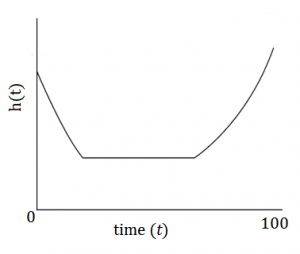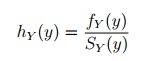# Hazard Function: Simple Definition

## What is a Hazard Function?The hazard function h(t) showing the chances of death for a human at any particular age.The hazard function (also called the force of mortality, instantaneous failure rate, instantaneous death rate, or age-specific failure rate) is a way to model data distribution in survival analysis. The most common use of the function is to model a participant’s chance of death as a function of their age. However, it can be used to model any other time-dependent event of interest.

More specifically, the hazard function models which periods have the highest or lowest chances of an event. The function is defined as the instantaneous risk that the event of interest happens, within a very narrow time frame. (Note: If you’re familiar with calculus, you may recognize that this instantaneous measurement is the derivative at a certain point).

Hazard functions and survival functions are alternatives to traditional probability density functions (PDFs). They are better suited than PDFs for modeling the types of data found in survival analysis.

## Conditional and Variations

The hazard function is a conditional failure rate, in that it is conditional a person has actually survived until time t. In other words, the function at year 10 only applies to those who were actually alive in year 10; it doesn’t count those who died in previous periods.

There are other variations on the function, other than as a conditional rate. The Kaplan Meier (KM) method uses rates, has no upper limit, and is preferred for clinical trials (Fink & Brown, 2006). Conversely, with the actuarial method, the hazard function is a proportion, with values between 0 and 1.

## Formula

The hazard function formula is:Where:

• fY(y) = the probability density function of survival time Y,
• SY = the Survivor function (the probability of surviving beyond a certain point in time)

## References

Der, G. & Everitt, B. (2007). Basic Statistics Using SAS Enterprise Guide: A Primer. SAS Institute.
Fink, S., Brown, R. (2006). Survival Analysis. Gastroenterol Hepatol (N Y). May; 2(5): 380–383. Retrieved May 28, 2018 from here (https://www.ncbi.nlm.nih.gov/pmc/articles/PMC5338193/).

CITE THIS AS:
Stephanie Glen. "Hazard Function: Simple Definition" From StatisticsHowTo.com: Elementary Statistics for the rest of us! https://www.statisticshowto.com/hazard-function/